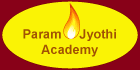Kindergarten:

• Writing
• Math : Numbers and Shapes

• Mathematics(Addition & Subtraction,Fraction,Geometry,Measurement,Numbers,Problem solving.Time and Money)
• Science
• Writing

• Mathematics ( Addition & Subtraction,Fractions,Numbers,Geometry,Graphing, Measurement, Multiplication and Division,Problem solving, Time and Money)
• Science
• English
• Writing

• Mathematics ( Addition & Subtraction,Fraction,Numbers, Geometry, Graphing, Measurements,Multiplication & Division, Problem solving,Time and Money)
• Science
• English
 Tomorrow Never Waits!Contact  Us Now.Gives confidence to your child to cope up with                              academic challenges.